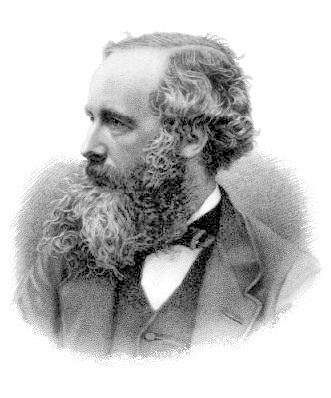﻿ Units: CGS and MKS

## CGS and MKS Units

In the middle of the nineteenth century scientists began to discuss how best to extend the metric system to include physical units such as force, pressure, work, and power. In order for this to be done in a coherent way, a choice must be made as to what metric units of distance, mass, and time should form the foundation of the system. A group of scientists including the Scottish mathematical physicist James Clerk Maxwell and the Scots-Irish physicist William Thomson (later ennobled as Baron Kelvin) were successful in arguing for the selection of the centimeter, gram, and second, and the resulting system is called the CGS System.

 The CGS system was introduced formally by the British Association for the Advancement of Science in 1874. It found almost immediate favor with working scientists, and it was the system most commonly used in scientific work for many years. But because the CGS units are relatively small in size, engineers preferred a system based on larger units. The MKS System, based on the meter, kilogram, and second, appeared within a few years as a competing choice for a coherent system of units. When we say, for example, that the dyne is the CGS unit of force, this determines its definition: it is the force which accelerates a mass of one gram at the rate of one centimeter per second per second. The MKS unit of force, the newton, is the force which accelerates a mass of one kilogram at the rate of one meter per second per second. Since the meter equals 100 centimeters and the kilogram equals 1000 grams, the ratio between a CGS unit and the corresponding MKS unit is characteristically a power of 10. Since a newton accelerates a mass 1000 times greater than a dyne does, and it does so at a rate 100 times greater, there are 100 000 = 105 dynes in a newton. During the 20th century, metric units based on the meter and kilogram--the MKS units--were used more and more in commercial transactions, engineering, and other practical areas. By 1950 there was some discomfort among users of metric units, because the need to translate between CGS and MKS units went against the metric ideal of a universal measuring system. In other words, a choice needed to be made. In 1954, the Tenth General Conference on Weights and Measures (CGPM) adopted the meter, kilogram, second, ampere, degree Kelvin, and candela as the basic units for all international weights and measures, and in 1960 the Eleventh General Conference adopted the name International System of Units (SI) for this collection of units. (The "degree Kelvin" became the kelvin in 1967.) In effect, these decisions gave the central core of the MKS system preference over the CGS system. Although some of the CGS units remain in use for a variety of purposes, they are being replaced gradually by the SI units selected from the MKS system.James Clerk Maxwell (1831-1879)

Following is a table of CGS units with their SI equivalents. Note that in some cases there is more than one name for the same unit. The CGS electromagnetic and electrostatic units are not included in this table, except for those which have special names.

 CGS unit measuring SI equivalent barye (ba) pressure 0.1 pascal (Pa) biot (Bi) electric current 10 amperes (A) calorie (cal) heat energy 4.1868 joule (J) darcy permeability 0.98692 × 10-12 square meter (m2) debye (D) electric dipole moment 3.33564 × 10-30 coulomb meter (C·m) dyne (dyn) force 10-5 newton (N) emu magnetic dipole moment 0.001 ampere square meter (A·m2) erg work, energy 10-7 joule (J) franklin (Fr) electric charge 3.3356 × 10-10 coulomb (C) galileo (Gal) acceleration 0.01 meter per second squared (m·s-2) gauss (G) magnetic flux density 10-4 tesla (T) gilbert (Gi) magnetomotive force 0.795 775 ampere-turns (A) kayser (K) wave number 100 per meter (m-1) lambert (Lb) luminance 3183.099 candelas per square meter (cd·m-2) langley heat transmission 41.84 kilojoules per square meter (kJ·m-2) line (li) magnetic flux 10-8 weber (Wb) maxwell (Mx) magnetic flux 10-8 weber (Wb) oersted (Oe) magnetic field strength 79.577 472 ampere-turns per meter (A·m-1) phot (ph) illumination 104 lux (lx) poise (P) dynamic viscosity 0.1 pascal second (Pa·s) stilb (sb) luminance 104 candelas per square meter (cd·m-2) stokes (St) kinematic viscosity 10-4 square meters per second (m2·s-1) unit pole magnetic flux 1.256 637 × 10-7 weber (Wb)